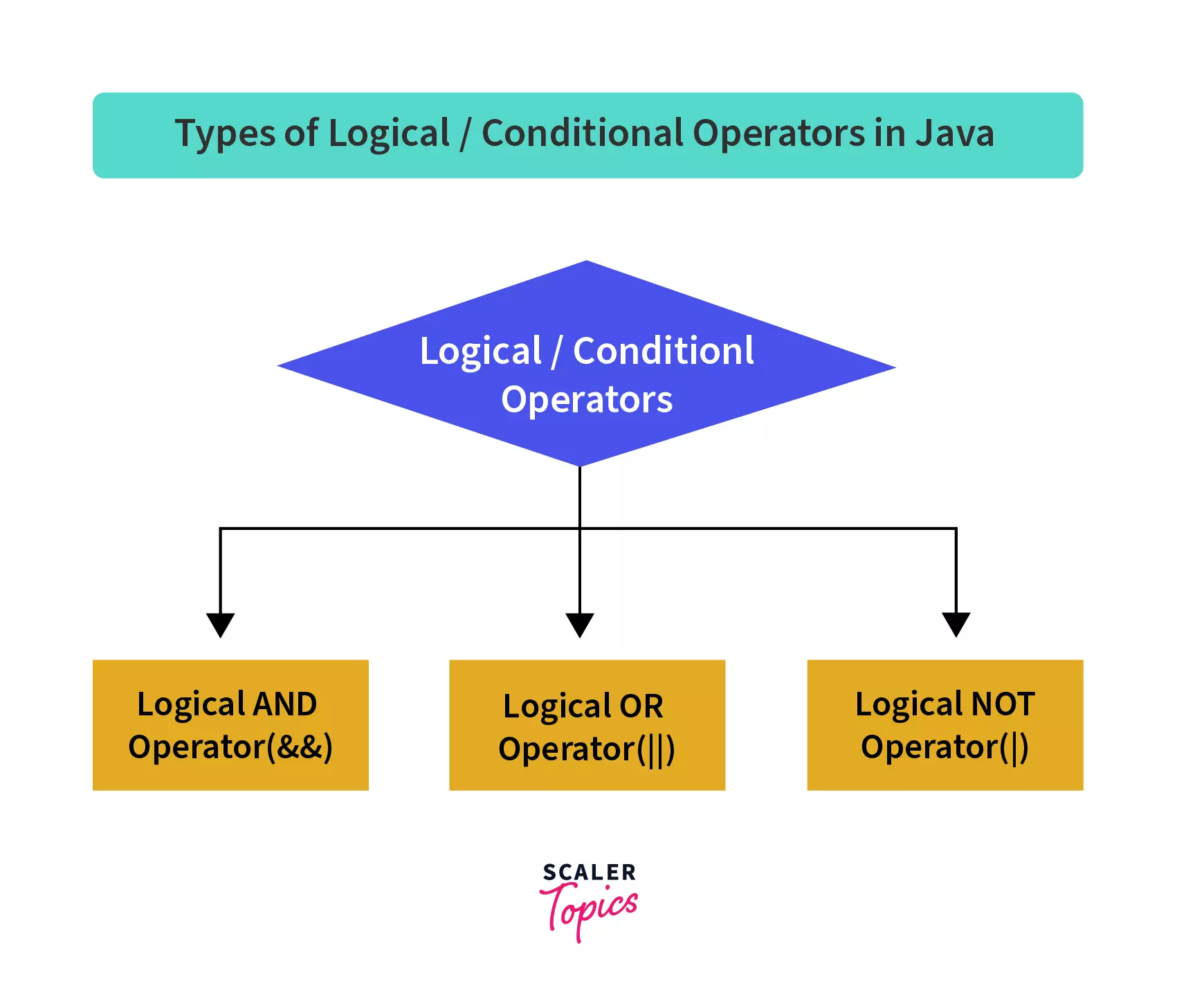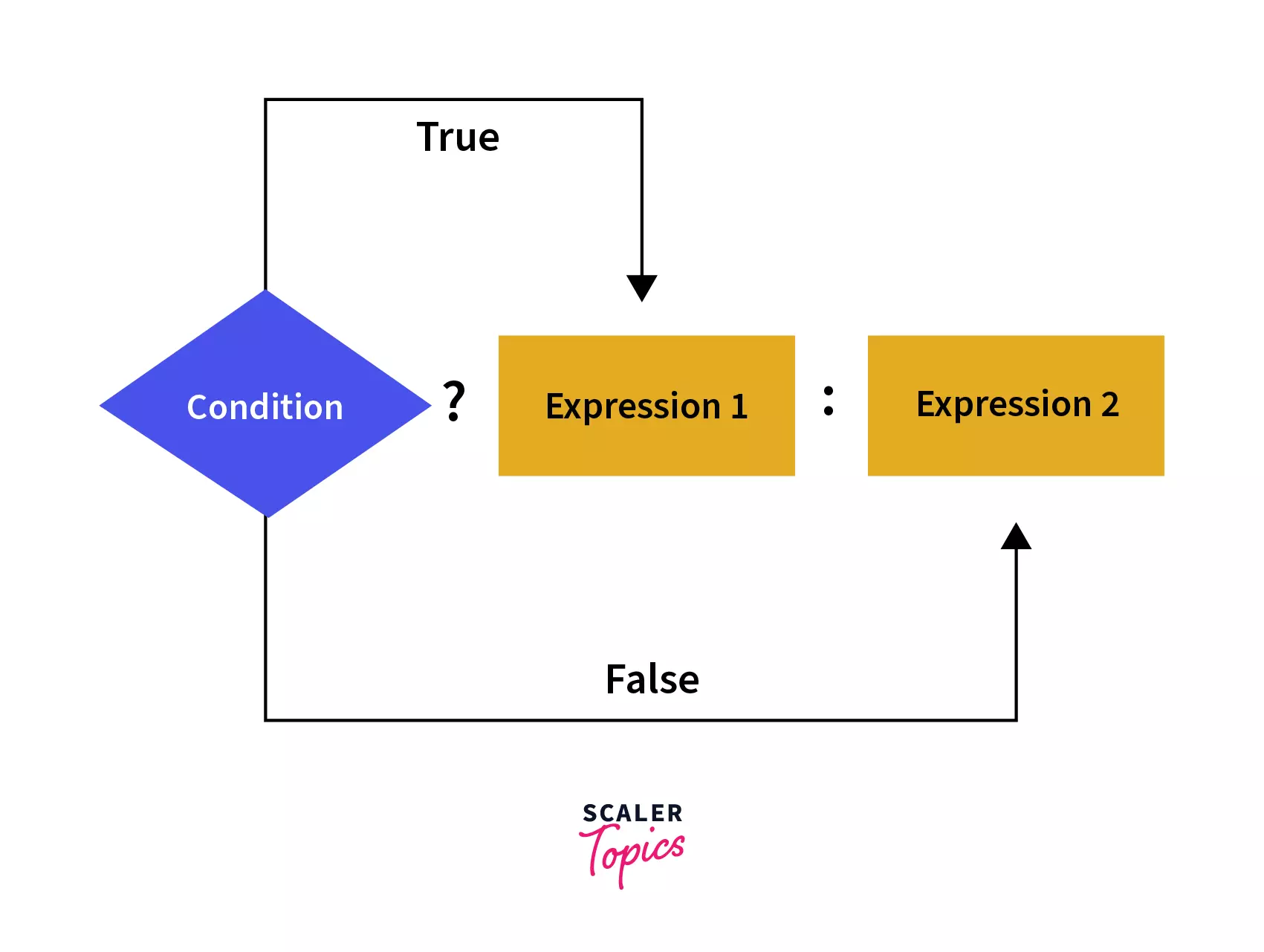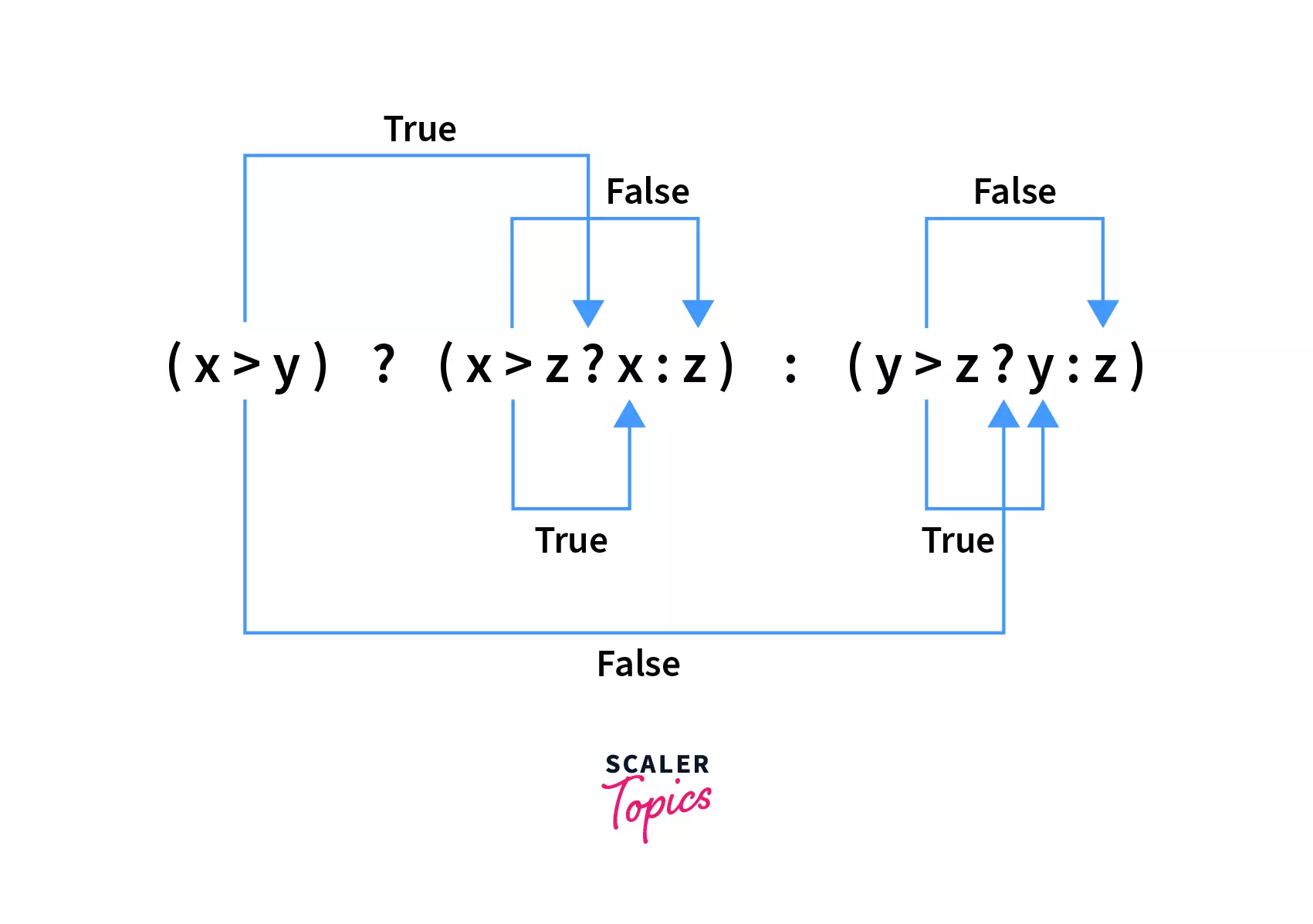# Conditional Operator in Java

Challenge Inside! : Find out where you stand! Try quiz, solve problems & win rewards!
Video Tutorial## Abstract

Conditional Operators are used in making decisions in programming just as we make decisions in real life. It controls the flow of the program and produces outcomes based on the provided conditions. There are three types of Conditional Operators called as Conditional AND, Conditional OR and Ternary Operator. Let's dig and find out how and when these conditional operators are used in java.

## Introduction to Conditional Operator in Java

Suppose you are given a number of regular polygons, you need to categorize it into its types. Three sided polygon into a triangle, four sided into a quadrilateral and so on. How do we execute these conditions and obtain correct output. Here comes the role of Conditional Operators in Java.

Conditional Operators in Java are used to work on conditions. It is a unique operator used in place of 'If-else' statements. They make complex code much easy to write and understand. How? you ask! Let's dive in and and find out.

## Three Types of Conditional Operator in java

There are three types of the conditional operators in Java:

1. Conditional AND
2. Conditional OR
3. Ternary Operator### Conditional AND (&&)

• Syntax: &&

Below is the table representing the output of AND operator depending on the value of expressions:

expr1expr2expr1 && expr2
TTT
TFF
FTF
FFF

Below is an example of using AND Operator in a programmatic way to get a better understanding:

In the above example, AND operator checks for both conditions (age and gender) and evaluates to true as both expressions yields true values and produces the output: "Teenage girl";

The best way to remember the output of AND operator is: The output is only true whenever both the expressions surrounding the operator are true.

### Conditional OR (||)

• Syntax: ||

Below is the table representing the output of OR operator depending on the value of expressions:

expr1expr2expr1 II expr2
TTT
TFT
FTT
FFF

Let's create a Java program and use the OR conditional operator to get a better understanding:

A student is only eligible for camp if he either built projects or scored marks greater than 70. Here is a logic to deduce if a student is eligible for camp or not.

In the above example, one expression yields true whereas other yields false. Taking OR of both expression returns true as per the table shown above. So the resultant output becomes: Eligibe for Camp

The best way to remember the output of OR operator is: The output is only true whenever either of the expressions surrounding the operator is true.

### Ternary Operator

The word ternary is the amalgamation of three parts. It is so named as it consists of 3 operands. Ternary operator is a condensed form of if-else statement which evaluates a condition and executes the code based on the evaluated condition. Let's get a clear understanding of how a ternary operator is written and used.

#### Teranry Operator Syntax:

result: The final variable which gets the value.

condition: This is the test condition statement that gets evaluated to true or false.

expression1: If condition gets evaluated to true then expression1 is assigned to result.

expression2 : If condition gets evaluated to false then expression 2 is assigned to result.

The only thing to remember when using ternary operation is: The very first operand must be a Boolean expression, and the second and third operands can be any expression that returns some value.If the above expression is written using If-else:

Example

Using Ternary Operator:

The above code checks the greater of the two variable and subtracts the smaller variable from greater one and stores the answer in result. Both code gave the same output. The use of Ternary Operator compressed the 4 line code into just 1 line making the code more comprehensive and readable.

## When to Use Ternary Operator

Though at byte code level Ternary Operator is not faster than if-else but it enhances the readability of code multiple times and makes it concise. Below are few examples how and when ternary operators can be used:

## Ternary Operator Example

### Example 1: Ternary operator as an alternative to if-else

Re-writing using Ternary Operator:

### Example 2: Ternary operator as an alternative to if-else-if

Re-writing using Ternary Operator:

### Example 3: Ternary operator as an alternative to switch-case

Let us consider a switch-case scenario.

Re-writing using Ternary Operator:

Hence ternary operator can be used to replace if-else, if-else-if as well as switch case statements making the code more clear and optimized.

## Expression Evaluation

When evaluating a ternary expression (condition) ? exp1: exp2. Only one of the two expressions, either exp1 or exp2 is evaluated at runtime. For instance if condition is true then exp1 gets evaluated otherwise exp2. Let's understand this with some examples.

Our Condition always evaluates to true so expression2 remained same and expression1 got incremented by 1.

Here expression1 remain unchanged and expression2 got incremented.

## Nesting Ternary Operator

Java allows the feature of Nesting ternary Opeartor. Nesting means using a ternary operator inside another ternary operator. Lets have a look at the code to understand more about nesting ternary operator.• In the above program, we evaluated the value of largest number using nested ternary operator.
• As illustrated in the diagram, the first expression (x>y) is evaluated and if it returns true then expression (x>z ? x : z) is evaluated else (y>z ? y : z) gets executed.
• If expression1 (x>z ? x : z) gets executed, then it again checks the condition (x>z) and returns value according to the condition.
• Similarly if expression2 (y>z? y : z) gets executed, then it checks for the condition (y>z) and returns the value according to the condition.
• After executing all expressions, result gets stored in largsetNum and it all gets done using just a single code of line! Interesting right!

## Ternary Operator as Null Check

Java ternary operator can also be used as a null check for an object before applying transformations to the object. Below is an example of how:

If null check for objects are not taken into considerations, NullPointerExceptions are generated in codes which can be avoided using ternary operator. In the above code, the obj reference is null therefore object.getValue() method is not called and it simply returns a null value.

## Ternary Operator as max Function

Java ternary operator comes in handy to achieve the same functionality as the Java Math max() function . Given below is an example of implementing Math.max() function using ternary operator.

The above code utilized the max() function present in Java library, which takes two parameters, num1 and num2 and returns the maximum of two given numbers. Since 20>10 we got 20 as the output. The numbers can be int, float, double and long.

Using ternary operator to find the maximum of two numbers:

We observed that ternary operator can easily be used in place of the inbuilt function to find the maximum of two numbers. Teranry Operator helps readers understand the inner working of code and how the logic is implemented to produce the correct output.

## Ternary Operator as Min Function

The ternary operator can also be used as a min function, i.e. to calculate the minimum of values same as java library min function, Math.min().

The above example demonstrated the use of min() function in Java. It also takes two parameters and returns the smaller of the two values . Here 10 is smaller than 20 so it produced 10 as its output.

Using ternary operator to find the minimum of two numbers:

Here also we can see that ternary operator can easily be used in place of the inbuilt function Math.min( ) to find the minimum of two numbers. Using ternary operator helped in**** understanding the logic behind the produced output more clearly.

## Ternary Operator as abs Function

Java Ternary Operator can also be used to implement Java Math abs() function. Here's an example.

## Benefits of Using Ternary Operator in Java

• It is used as shorthand for if-else and switch-case statements.
• It produces a comprehensive program with reduced number of lines of programming.
• It makes the code more clear, concise and readable by scaling down the effective number of lines.
• It can be nested to provide conditions within other conditions.
• The ternary operator enables a form of "optional" parameter to easily inline a default choice when null is supplied for a parameter value.

## Conclusion

• Conditional Operators are used to work on conditions in Java. They control the flow of a program.
• Ternary Operator is used in place of if-else, if-elseif and switch-case statements.
• Ternary Operator enhances the conciseness and readability of code.

## FAQs

Q. Does the condition of a Java Ternary operator always evaluates a boolean value?

A. Yes, the condition of a Java Ternary operator always evaluates to either true or false.

Q. Can the Ternary operator be used in place of if-else statements?

A. Yes, Ternary operators are used as shorthand for if-else statements.

Q. Does Java allows nested ternary operator?

A. Yes, Java allows the feature of the Nesting ternary operator.